# Troubleshooting sensor wiring

Subjects:

• Preface
• Measurement without interference
• Fault 1 – Interrupted signal wire
• Fault 2 – Open Supply Wire
• Fault 3 – Interrupted ground wire
• Fault 4 – Transition Resistance
• Fault 5 – Short circuit between power and signal wire
• Fault 6 – Short circuit between power and ground wire
• Fault 7 – Short circuit in sensor C
• Fault 8 – No power supply due to defective ECU
• Fault 9 – Interrupted PWM Signal Wire
• Repair of an interrupted plus wire

Preface:
If we suspect that there is a malfunction, we first read out the car. The fault code gives us a direction to look further. If no error codes are stored in the error memory, we will see if we can recognize deviations in the live data. See the page On Board Diagnostics.

Just because the error code is related to a sensor, that doesn't mean the sensor is faulty. To rule out whether there is a problem in the wiring and/or plug connections, check with the help of electrical diagrams and measuring equipment exclude certain things. This page outlines a number of possible situations and shows that the description of the fault code may differ from the actual cause.

Measurement without interference:
The following figure shows the measurement of the supply voltage and ground of an active sensor.

The active sensor receives a plus (5 volts) and a ground via the control unit. In this case, the power supply is in order. We can do a second measurement on the ground wire (pin 3 on the sensor, and/or pin 4 on the ECU). The signal must be between 0,5 and 4,5 volts.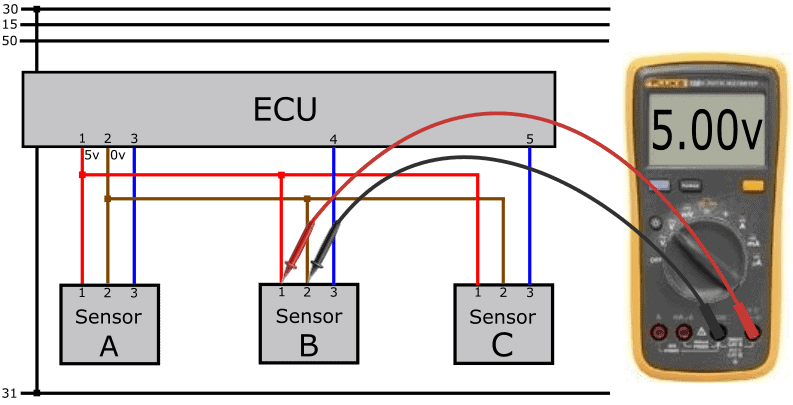The sensor uses the supply voltage of 5 volts to form the signal. The signal must be between 0,5 and 4,5 volts. The ECU reads the voltage level (or in other cases the frequency) and translates this into a value. For example, this could be the value of the boost pressure sensor are: at a turbo pressure of 1,5 bar, the sensor sends a voltage of 3,25 volts to the ECU.

In this measurement, the signal voltage with respect to ground is measured and is OK.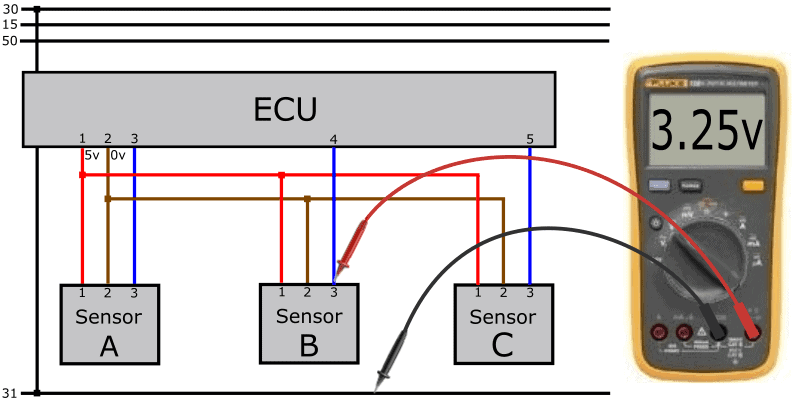With help from a breakout box we can measure in the plug of the ECU. We then know which voltages the ECU sends and receives.

In the next measurement we measure 3,25 volts again, but at the input of the ECU. This means that the signal wire is OK: the voltage is transmitted 1:1 from the sensor to the ECU.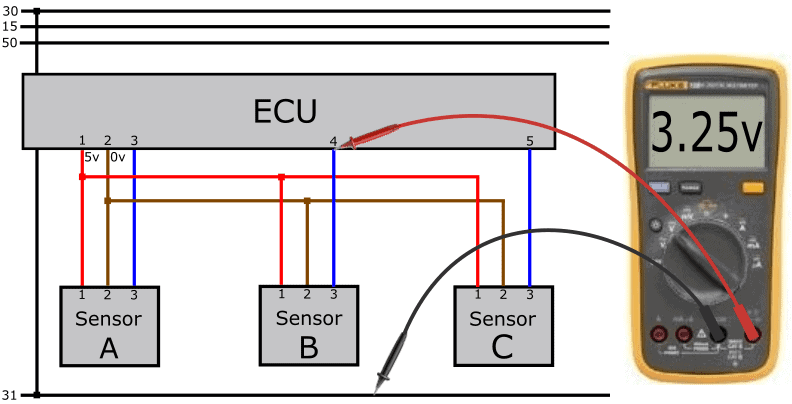The sensor signal will never be 0,0 or 5,0 volts. A certain range is always maintained. This is often between 0,5 and 4,5 volts. The sensor will not output voltages lower than 0,5, or higher than 4,5 volts. In case of defects in the sensors or the wiring, the ECU can recognize from the level of the voltage whether the value falls within or outside the measuring range:

• voltages less than 0,5 volts: the ECU generates an error code with the description: “sensor X, short circuit to ground” or “ground short”;
• voltages above 4,5 volts indicate “positive close” in the fault code description.

Active sensors can also send a digital signal. Usually these sensors are not powered by the ECU, but via terminal 15. In most cases we are dealing with a PWM signal.

The following image shows part of the schematic where an active sensor has an external power supply and the signal wire is connected via pin 3 of the sensor to pin 4 of the ECU. The voltage curve of the sensor with respect to the ground connection is measured with the oscilloscope.

The scope is set to 2 volts and 5 milliseconds per division. The duty cycle is 50%.

In the paragraph: Fault 9 – Interrupted PWM Signal Wire we will discuss the steps to make a proper diagnosis.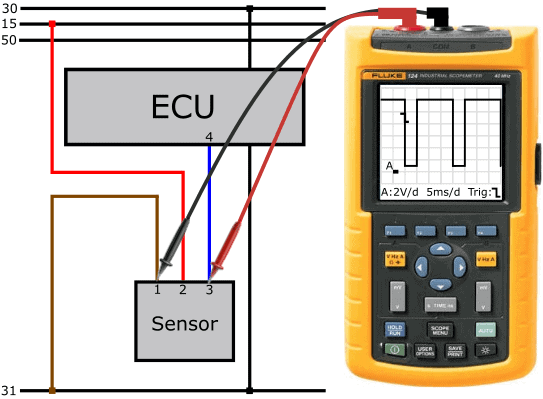Diagnosing the sensor wiring:
Before diagnosing sensors, we need to be aware of the type of sensor (passive, active, intelligent) and how the sensor sends its signal to the control unit (analog or digital, in the form of an AM ( Amplitude Modulation) or FM (Frequency Modulation) After consulting the electrical diagram, we can estimate which voltages we are going to measure on the wiring.

The following paragraphs outline possible malfunctions that can occur in practice. Instead of starting with “the customer's complaint”, the cause is immediately mentioned; eg: an interrupted wire, short circuit, etc. This is about gaining insight into the measurement techniques. Because how do you act in the event of a malfunction? And with which measurements do you find out the cause?

Do you master the measurement techniques and are you curious about a case? Then visit the page: Case: fuel pressure sensor failure short circuit to plus.

Fault 1 – Interrupted signal wire:
With an interrupted signal wire, the signal voltage from the sensor cannot reach the ECU. In this section you can read what you measure in this situation on the connections of both the sensor and the ECU.

We perform the following measurements on the active sensor and get the following readings:

• supply wire (pin 1) to sensor ground (pin 2) is 5 volt;
• signal voltage to ground is 2,9 volt.

The power supply and the generated sensor signal are OK. However, the sensor signal does not reach the ECU due to the interruption.
To measure the voltage at the input of the ECU, we use a breakout box.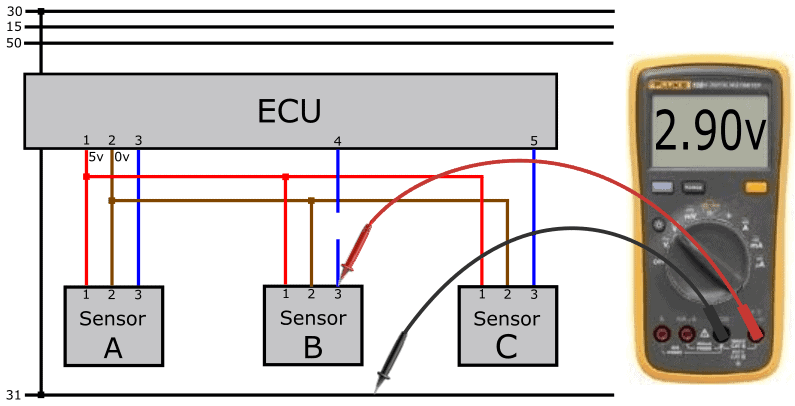Using a breakout box, we perform a measurement on pin 4 of the ECU with respect to ground (or pin 2 of the sensor). We measure a voltage of 4,98 volts.

The voltage on the ECU side is therefore higher than the voltage sent by the sensor. A circuit in the ECU is responsible for the output voltage of 4,98 volts. On the one hand, this has to do with the way of signal processing, but also with regard to recognizing interruptions.

The ECU now measures its own output voltage and recognizes this as a positive circuit due to the supply voltage of 4,98 volts.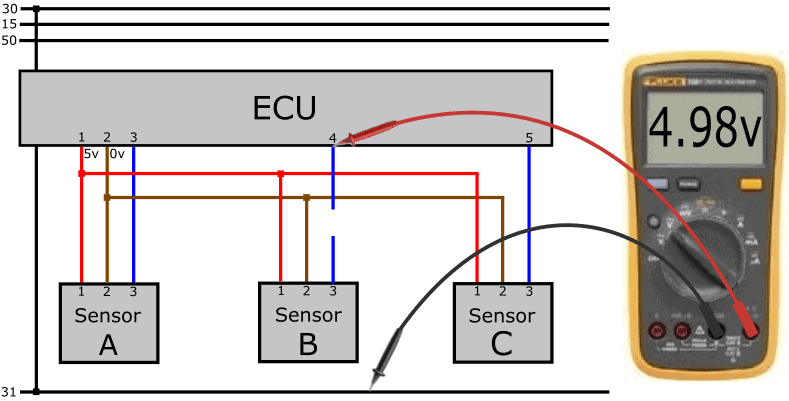We then measure the voltage difference across the wire between the ECU and the sensor. The voltage difference should be close to 0 volts in a fault-free situation.

In this case we measure a voltage difference of 2,08 volts; namely 2,9 volts (sensor) versus 4,98 volts (ECU).Remove the plug from the sensor. If there was no break in the wire, we would measure the 4,98 volts from the ECU in the removed plug. Now we measure 4 volts on pin 4,98 of the ECU, but 0 volts in the removed plug.

In this case we can already conclude that the signal wire is interrupted.With an interrupted signal wire, the voltage on the signal input of the ECU is approximately 5.0 volts. On the page: sensor types and signals, in the paragraph: “voltage supply and signal processing” you can read how the ECU processes the signal from the active sensor. With that knowledge you can better understand how we can deal with disturbances such as the interrupted signal wire.

The voltage of 4,98 volts is generated in the ECU. Between the positive wire (from the 78L05) and the ADC are a number of resistors that pull the signal voltage to 5 volts when no voltage comes in via the signal connection. The ADC measures this voltage and processes this voltage into a digital signal. Thus, the ECU receives a signal over a voltage that is out of range and generates an error code.

Please note: with a similar malfunction, the voltage is not always exactly 4,98 or 5,0 volts!
On the page: Case: fuel pressure sensor failure – short circuit to plus a fault is described where this voltage value deviates.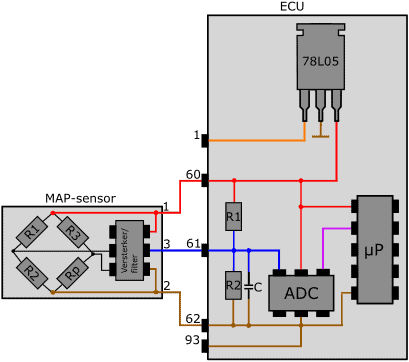Fault 2 – Interrupted Power Wire:
There is an interruption between the plus wire junction between the three sensors and the sensor plug. The supply voltage of 5 volts cannot reach the sensor now. The sensor cannot function without a power supply and ground.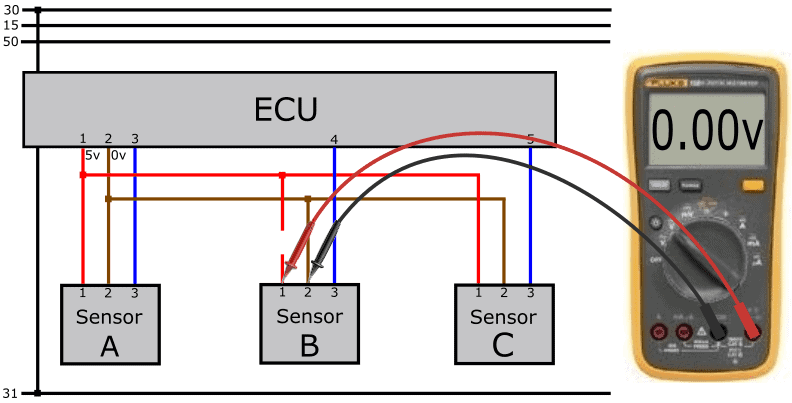Because we measured the power and ground on the plug in the previous measurement, we still have to rule out which of the two wires has a problem. We therefore measure the plus on another sensor in the same plus circuit. It is of course also possible to use the ECU if a breakout box is available.

On pin 1 of sensor A relative to the ground of sensor B we measure 5 volts. This means that the mass of sensor B is OK.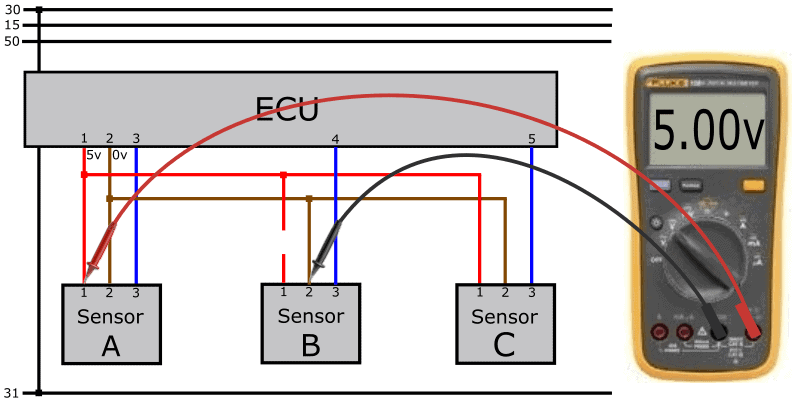When no current flows through the electronics of the active sensor through the interrupted power wire, we measure a voltage of 4,98 volts on the signal input of the ECU. We have a similar situation as with the broken signal wire: the internal resistors in the ECU pull the signal voltage up to 4,98 volts. Because the signal wire is OK in this case, we also measure the voltage of 4,98 volts on the plug of the sensor.In the cases where the voltage is just above 5,0 volts, the voltage of the voltage stabilizer may have been lifted. See the paragraph: “voltage supply and signal processing” on the page: “sensor types and signals".

Fault 3 – Interrupted ground wire:
In this case, not the plus, but the ground wire is interrupted. A supply voltage of 5 volts does arrive at the sensor, but because we are measuring relative to an interrupted wire, the voltmeter has no reference voltage and shows 0 volts.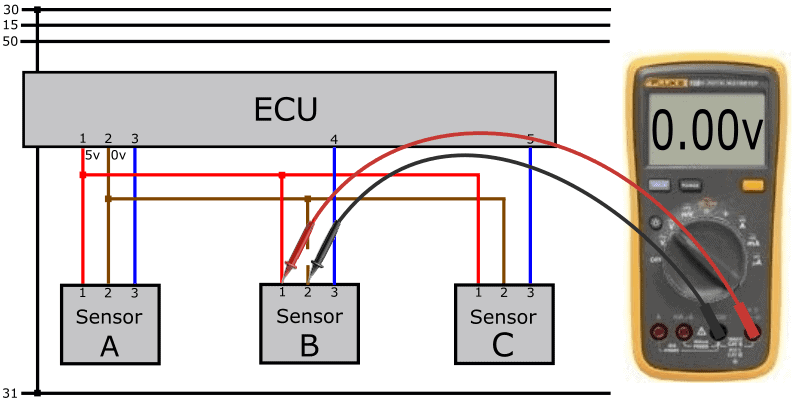When moving the minus measuring pin to the ground of the bodywork or the battery, the voltmeter shows 5 volts.

When we connect the minus test pin to the ground connection of sensors A and C, we also need to measure 5 volts difference. If we were to measure 2 volts in pin 5 of sensor A, but not 5 volts at sensor C, the interruption would be in the wire between sensor A and B, so between the first two nodes.

As with the interrupted signal and positive wire, we now measure a voltage of 4,98 volts on the signal wire.Fault 4 – Transition Resistance:
In the previous section, voltage loss as a result of a contact resistance was already discussed. In the following diagram we see a resistance in the supply wire. The moment current flows through the supply wire, the transition resistance causes a (presumably) too low voltage on pin 1 of plug B. We measure 4 volts instead of the 5 volts we expected to measure.

The stored DTC description can be in this case: “Signal lower limit value undershot”.If we measure pin 1 of connector B versus pin 1 of connector C, we should have a difference of (5-5) = 0 volts. We now see 1 volt difference.

Since the voltage drop is only present in the wire of sensor B and not of sensor C, we can assume that the wire between the node of the horizontal wire in the diagram and the plug is not OK.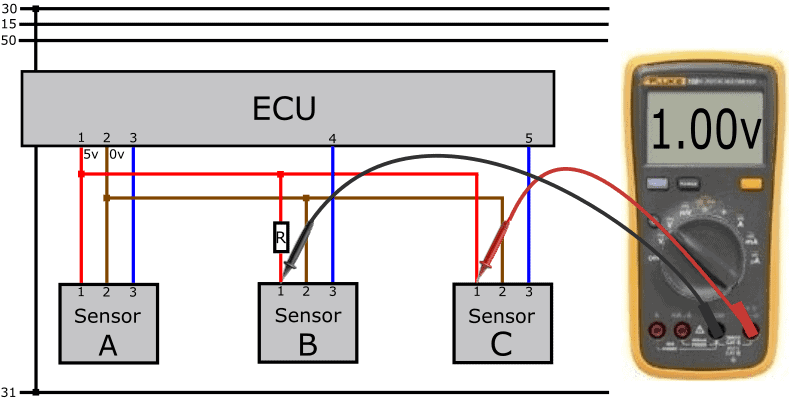Fault 5 – Short circuit between supply and signal wire:
A possible fault in the wiring is a short circuit. We encounter a short circuit in the following situations:

• between the power supply wire and the signal wire (plus terminal);
• between the ground wire and the signal wire (ground fault);
• between one of the three wires to each other and/or to the body (ground connection);

In this diagram we see a short circuit between the signal wire and the positive wire (plus circuit). We measure a signal voltage equal to the supply voltage of 5 volts.When measuring 5 volts on pin 3 of the sensor and pin 4 of the ECU, the problem may be internal to the sensor. To rule that out, we check for short circuits in the wiring with an Ohm meter. To obtain a safe and correct measurement, we switch off the ECU, remove the plug from the ECU and remove the plugs from the sensors that are connected to each other with the nodes. Because there is a short circuit, we measure a connection with the Ohm meter.In this case it is 0,0 Ohm because the wires connect to each other. In reality, this value can be a few Ohms higher. When no short is present, the ohmmeter will indicate OL or 1. (infinite resistance) because there is no electrical connection between the leads and probes.

Fault 6 – Short circuit between supply and ground wire:
In the event of a short circuit between the power and ground wires, the ECU switches off the power of pin 1. All sensors powered by pin 1 will no longer function. Thus, trouble codes will be stored across multiple sensors.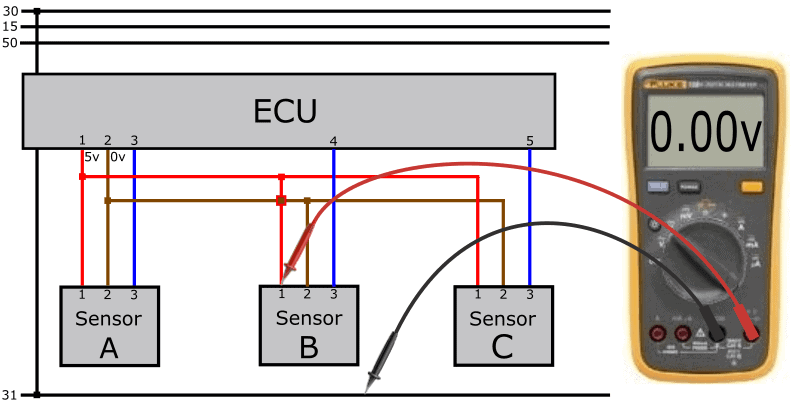In this case we also measure a voltage of 5,0 on the signal wire, which comes from the ECU.

To rule out whether we are dealing with a short circuit, we disassemble the plugs of both the ECU and all sensors in the circuit concerned, just like in the previous section. Use an ohmmeter to measure the resistance between the red and brown wires.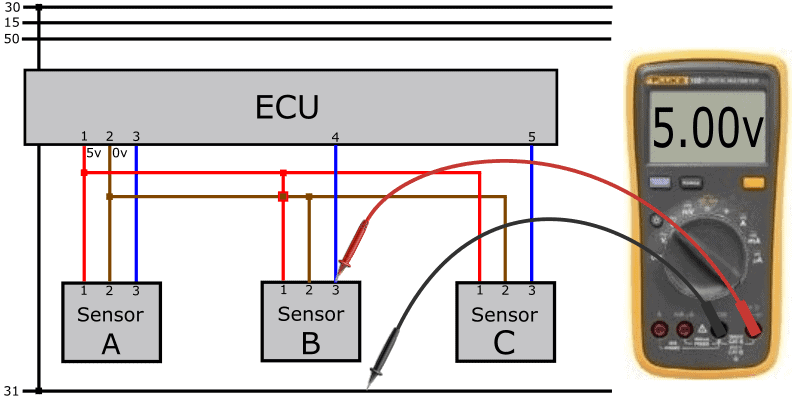Fault 7 – Short circuit in sensor C:
When measuring the supply voltage in relation to the ground, we again measure 0 volts. In the previous outage we had a short circuit in the wiring. In this case, the short circuit is internal to a sensor.

One by one we disconnect the plugs from the sensors that we see in the diagram. When removing the plug from sensor C, we no longer have a short circuit, and the ECU will supply the plus wire with 5 volts again. With some versions this is done automatically, with other types a clamp change is required.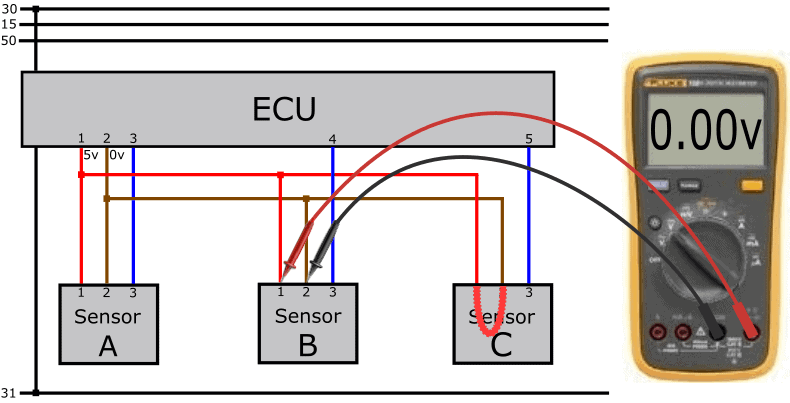Fault 8 – No power supply due to faulty ECU:
In a few cases it can happen that the ECU is the culprit of the missing power supply. Internally, a circuit is damaged and 5 volts are not being sent out.

The ECU is often incorrectly reported as defective. In most cases there is another cause. Therefore, first check the possible interruptions and short circuits in the wiring and the connected sensors. To rule out whether an internal defect in the ECU is the cause, we check all ground connections of the ECU.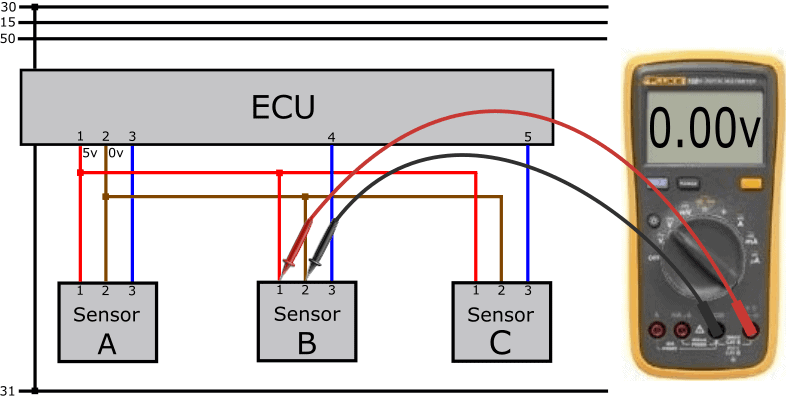With an extensive engine management system we see several circuits in an ECU, each with their own ground wire. We sometimes find as many as eight ground wires in one plug. The moment that one pin in the plug makes poor contact, or one ground wire in the wiring harness has an interruption, that circuit will shut down. Therefore, preferably in a loaded condition, measure with a test lamp (plus on the battery, minus on each ground connection in the plug of the ECU) whether the ground is OK. The test lamp must light up equally brightly on each ground wire. Does the lamp not light up with one ground connection? Then you may have identified the cause and the ECU is not defective.

Fault 9 – Interrupted PWM Signal Wire:
So far we have talked about analog voltages that can be measured with a multimeter. In case it is a digital signal, a multimeter is no longer sufficient. We then use the oscilloscope. The following text is about the oscilloscope in the images below. Here we see the Fluke 124 with a modified screen display.

The reason for performing this measurement is the fault description that can be translated from the fault code. The description reads: “sensor signal interrupted”.

The scope image shows a constant voltage line of 0 volts. This means that there is no voltage difference between the test probes. Have you measured that the plus and ground wires of the sensor are good (pin 2 to 1), in this case around 13 volts, there is something wrong with the signal wire. Note that the sensor can transmit the information in two ways:

1. The sensor sends a positive voltage to the ECU (usually an analog voltage;
2. The ECU sends a voltage, which is connected to ground by the sensor on a time basis (by means of PWM; a digital signal).

In the example, the signal voltage on the sensor side is 0 volts, so we're going for way 2.

Because the signal wire is interrupted, the sensor does not receive voltage from the ECU.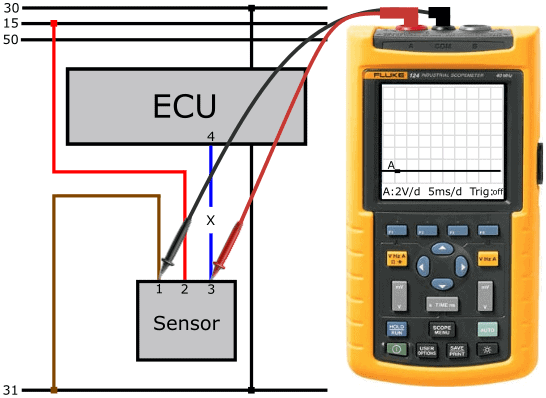We measure pin 4 of the ECU relative to pin 1 of the plug. The voltage is 12 volts. With these measurements, we have determined that the sensor input of the ECU is OK.

The ECU is apparently sending a constant voltage, but it is not reaching the sensor. The sensor therefore has no voltage to connect to ground.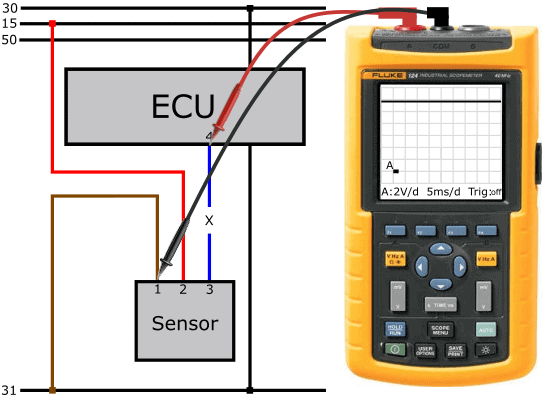At the next measurement, we connect the measuring pins on both sides of the signal wire. With this we determine the voltage difference in active state across the wire. The voltage must be 0 volts in a fault-free situation. However, at the active part of the square-wave voltage we see a voltage of 12 volts. When we get the complete If we measure the supply voltage in the maximum positive part of the square-wave voltage, then in most cases we are dealing with an interrupted wire. This is now also the case: the output voltage of the ECU (pin 4 to ground) is 12 volts.

Furthermore, in the lower part of the square-wave voltage a deviation: dThe voltage line drops to about 5 volts, remains constant for 10 milliseconds with a ripple, then rises again to 12 volts. Because the oscilloscope is now in series between the pull-up resistor in the ECU and the pull-down resistor in the sensor, a series connection is created. The scope has a high internal resistance, which affects the signal. The signal is not usable for this reason.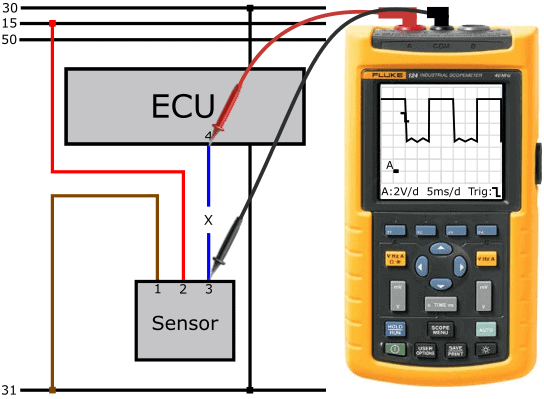Although the load voltage measurement is sufficient for a good diagnosis, it does not hurt to show with a resistance measurement that there is actually a broken connection in the wire. In this case we measure an infinitely high resistance (OL or 1.)

After the repair of the signal wire, we again measure signal voltage with respect to ground. Note: we are measuring here relative to ground, so the "active" part of the sensor in the PWM signal is now reversed...
We see in this scope image that:

• the voltage is a maximum of 12 volts. Here the sensor is not active: the voltage on the signal wire is not drawn to ground.
• the voltage drops to 1 volt. The sensor is active here: the sensor puts the voltage from the ECU to ground via the sensor electronics.

The sensor contains an electronic circuit that still uses 1 volt. With this voltage, the ECU can also recognize that the sensor switches on properly. The ECU can determine from the voltage levels whether the sensor is functioning properly:

• voltage over an extended period of time is equal to or greater than 12 volts:
ECU recognizes an interruption or plus closure;
• voltage lower than 1 volt: ECU detects a ground fault.Repair of an interrupted positive wire:
Of the five faults described in the previous paragraphs, they can in most cases be remedied fairly easily.

Cut the wire from the wire with the break or contact resistance as short as possible in the wiring harness.
Apply insulation if necessary. Locate the closest sensor connected in the same power circuit. With active sensors you can easily find this in an electrical diagram. In the schematic, the closest sensor is C. Neatly solder a new wire to the plus wire.

Always work with heat shrink tubing to avoid future problems due to moisture ingress. If you seal this with insulating tape, new problems will arise in the foreseeable future!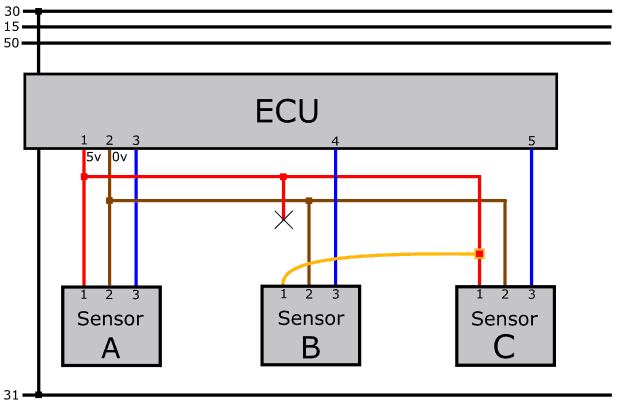error: Alert: Content is protected !!